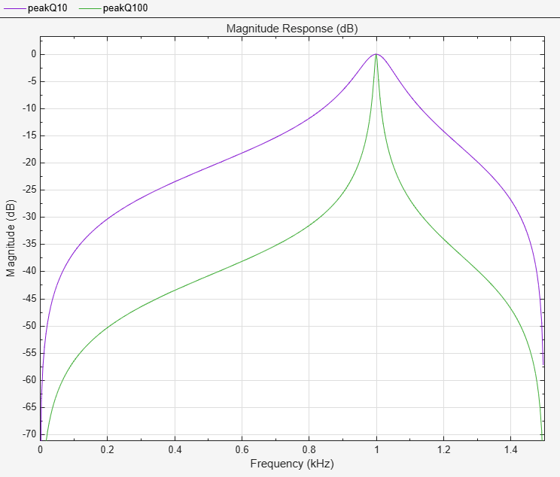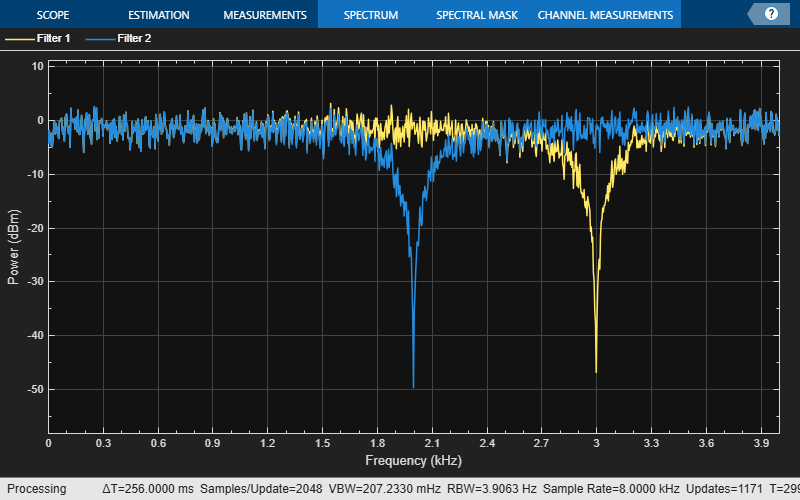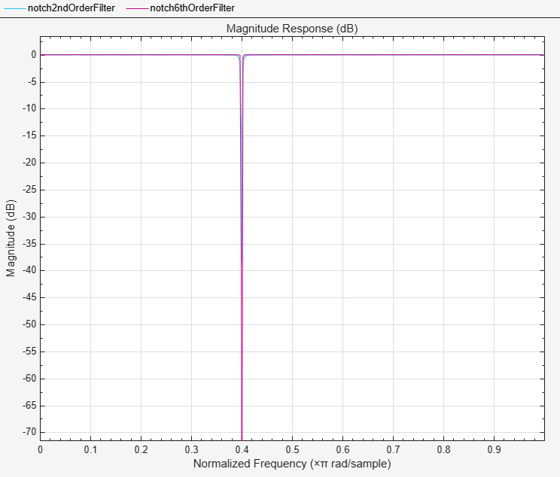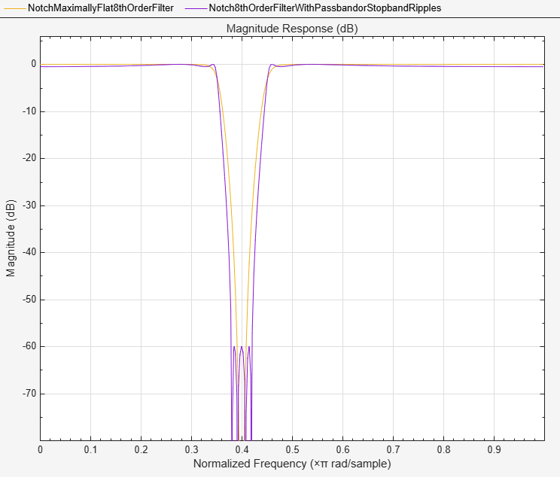Main Content

# Design of Peaking and Notching Filters

This example shows how to design peaking and notching filters. Filters that peak or notch at a certain frequency are useful to retain or eliminate a particular frequency component of a signal. The design parameters for the filter are the frequency at which the peak or notch is desired, and either the 3-dB bandwidth or the filter's Q-factor. Moreover, given these specifications, by increasing the filter order, it is possible to obtain designs that more closely approximate an ideal filter.

### Notch Filters

Suppose you need to eliminate a 60 Hz interference in a signal sampled at 3000 Hz. A notch filter can be used for such purpose.

```F0 = 60; % interference is at 60 Hz Fs = 3000; % sampling frequency is 3000 Hz notchspec = fdesign.notch('N,F0,Q',2,F0,10,Fs); notchfilt = design(notchspec,'SystemObject',true); fvtool(notchfilt,'Color','white');```The quality factor or Q-factor of the filter is a measure of how well the desired frequency is isolated from other frequencies. For a fixed filter order, a higher Q-factor is accomplished by pushing the poles closer to the zeros.

```notchspec.Q = 100; notchfilt1 = design(notchspec,'SystemObject',true); fvt= fvtool(notchfilt, notchfilt1, 'Color','white'); legend(fvt,'Q = 10','Q = 100');```An equivalent way of specifying the quality factor it to specify the 3-dB bandwidth, BW. They are related by Q = F0/BW. Specifying the bandwidth may be a more convenient way of achieving exactly the desired shape for the filter that is designed.

```notchspec = fdesign.notch('N,F0,BW',2,60,5,3000); notchfilt2 = design(notchspec,'SystemObject',true); fvt= fvtool(notchfilt, notchfilt1, notchfilt2, 'Color','white'); legend(fvt,'Q = 10','Q = 100','BW = 5 Hz');```Since it is only possible to push the poles so far and remain stable, in order to improve the brickwall approximation of the filter, it is necessary to increase the filter order.

```notchspec = fdesign.notch('N,F0,Q',2,.4,100); notchfilt = design(notchspec,'SystemObject',true); notchspec.FilterOrder = 6; notchfilt1 = design(notchspec,'SystemObject',true); fvt= fvtool(notchfilt, notchfilt1, 'Color','white'); legend(fvt,'2nd-Order Filter','6th-Order Filter');```For a given order, we can obtain sharper transitions by allowing for passband and/or stopband ripples.

```N = 8; F0 = 0.4; BW = 0.1; notchspec = fdesign.notch('N,F0,BW',N,F0,BW); notchfilt = design(notchspec,'SystemObject',true); notchspec1 = fdesign.notch('N,F0,BW,Ap,Ast',N,F0,BW,0.5,60); notchfilt1 = design(notchspec1,'SystemObject',true); fvt= fvtool(notchfilt, notchfilt1, 'Color','white'); legend(fvt,'Maximally Flat 8th-Order Filter',... '8th-Order Filter With Passband/Stopband Ripples', ... 'Location','NorthWest'); axis([0 1 -90 0.5]);```### Peak Filters

Peaking filters are used if we want to retain only a single frequency component (or a small band of frequencies) from a signal. All specifications and tradeoffs mentioned so far apply equally to peaking filters. Here's an example:

```N = 6; F0 = 0.7; BW = 0.001; peakspec = fdesign.peak('N,F0,BW',N,F0,BW); peakfilt = design(peakspec,'SystemObject',true); peakspec1 = fdesign.peak('N,F0,BW,Ast',N,F0,BW,80); peakfilt1 = design(peakspec1,'SystemObject',true); fvt= fvtool(peakfilt, peakfilt1, 'Color','white'); legend(fvt,'Maximally Flat 6th-Order Filter',... '6th-Order Filter With 80 dB Stopband Attenuation','Location','North');```### Time-Varying Notch Filter Implementations

Using time-varying filters requires changing the coefficients of the filter while the simulation runs. To complement the automatic filter design workflow based on fdesign objects, DSP System Toolbox provides other capabilities, including functions to compute filter coefficients directly, e.g. iirnotch

A useful starting point is a static filter called from within a dynamic (streamed) simulation. In this case a 2nd-order notch filter is created directly and its coefficients computed with iirnotch. The design parameters are a 1 kHz center frequency and a 50 Hz bandwidth at -3 dB with a 8 kHz sampling frequency.

```Fs = 8e3; % 8 kHz sampling frequency F0 = 1e3/(Fs/2); % Notch at 2 kHz BW = 500/(Fs/2); % 500 Hz bandwidth [b, a] = iirnotch(F0, BW)```
```b = 1×3 0.8341 -1.1796 0.8341 ```
```a = 1×3 1.0000 -1.1796 0.6682 ```
```biquad = dsp.BiquadFilter('SOSMatrix', [b, a]); scope = dsp.SpectrumAnalyzer('PlotAsTwoSidedSpectrum', false, ... 'SampleRate', Fs, ... 'SpectralAverages', 16); samplesPerFrame = 256; nFrames = 4096; for k = 1:nFrames x = randn(samplesPerFrame, 1); y = biquad(x); scope(y); end```The coefficients of time-varying filters need to change over time due to runtime changes in the design parameters (e.g. the center frequency for a notch filter). For each change of the design parameters, the coefficient vectors b and a need to be recomputed and biquad.SOSMatrix set to a new value. This can be computationally expensive. For this type of application dsp.CoupledAllpassFilter may offer more convenient filter structures. The advantages include - Intrinsic stability - Coefficients decoupled with respect to design parameters

Build a coupled allpass lattice filter equivalent to biquad

`[k1, k2] = tf2cl(b, a) %#ok`
```k1 = [] ```
```k2 = 2×1 -0.7071 0.6682 ```
```apnotch = dsp.CoupledAllpassFilter('Lattice', k1, k2); fvtool(apnotch, 'Fs', Fs)```One benefit of this allpass-based structure is that the new coefficients are decoupled with respect to the design parameters F0 and BW. So for instance a change of F0 to 3 kHz would yield

```F0 = 3e3/(Fs/2); [b, a] = iirnotch(F0, BW)```
```b = 1×3 0.8341 1.1796 0.8341 ```
```a = 1×3 1.0000 1.1796 0.6682 ```
`[k1, k2] = tf2cl(b, a)%#ok`
```k1 = [] ```
```k2 = 2×1 0.7071 0.6682 ```

NOTE: while a and b both changed, in the lattice allpass form the design change only affected k2(1).

Now change the bandwidth to 1 kHz

```BW = 1e3/(Fs/2); [b, a] = iirnotch(F0, BW)```
```b = 1×3 0.7071 1.0000 0.7071 ```
```a = 1×3 1.0000 1.0000 0.4142 ```
`[k1, k2] = tf2cl(b, a)%#ok`
```k1 = [] ```
```k2 = 2×1 0.7071 0.4142 ```

The design change now only affected k2(2).

Coefficient decoupling has numerous advantages in real-time systems, including more economical coefficient update and more predictable transient behaviour when coefficients change.

The following applies the above principle to changing the design parameters during a dynamic simulation, including the live visualization of the effects on the estimation of the filter transfer function. In real-world applications, one would generally identify the individual expressions that link each design parameters to the corresponding lattice allpass coefficient. Using those instead of filter-wide functions like iirnotch and tf2cl within the main simulation loop would improve efficiency.

```% Notch filter parameters - how they vary over time Fs = 8e3; % 8 kHz sampling frequency f0 = 1e3*[0.5, 1.5, 3, 2]/(Fs/2); % in [0, 1] range bw = 500/(Fs/2) * ones(1,4); % in [0, 1] range myChangingParams = struct('F0', num2cell(f0), 'BW', num2cell(bw)); paramsChangeTimes = [0, 7, 15, 20]; % in seconds % Simulation time management nSamplesPerFrame = 256; tEnd = 30; nSamples = ceil(tEnd * Fs); nFrames = floor(nSamples / nSamplesPerFrame); % Object creation apnotch = dsp.CoupledAllpassFilter('Lattice'); scope = dsp.SpectrumAnalyzer('PlotAsTwoSidedSpectrum', false, ... 'SampleRate', Fs, ... 'SpectralAverages', 4, ... 'RBWSource', 'Auto'); paramtbl = ParameterTimeTable('Time', paramsChangeTimes, ... 'Values', myChangingParams, ... 'SampleRate', Fs/nSamplesPerFrame); % Actual simulation loop for frameIdx = 1:nFrames % Get current F0 and BW [params, update] = paramtbl(); if(update) % Recompute filter coefficients if parameters changed [b, a] = iirnotch(params.F0, params.BW); [k1, k2] = tf2cl(b, a); % Set filter coefficients to new values apnotch.LatticeCoefficients1{1} = k1; apnotch.LatticeCoefficients2{1} = k2; end % Generate vector of white noise samples x = randn(nSamplesPerFrame, 1); % Filter noise y = apnotch(x); % Visualize spectrum scope(y); end```## SupportGet trial now